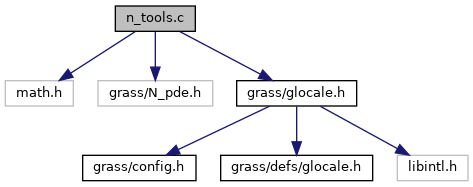GRASS GIS 7 Programmer's Manual  7.9.dev(2021)-e5379bbd7
n_tools.c File Reference
`#include <math.h>`
`#include <grass/N_pde.h>`
`#include <grass/glocale.h>`
Include dependency graph for n_tools.c:Go to the source code of this file.

## Functions

double N_calc_arith_mean (double a, double b)
Calculate the arithmetic mean of values a and b. More...

double N_calc_arith_mean_n (double *a, int size)
Calculate the arithmetic mean of the values in vector a of size n. More...

double N_calc_geom_mean (double a, double b)
Calculate the geometrical mean of values a and b. More...

double N_calc_geom_mean_n (double *a, int size)
Calculate the geometrical mean of the values in vector a of size n. More...

double N_calc_harmonic_mean (double a, double b)
Calculate the harmonical mean of values a and b. More...

double N_calc_harmonic_mean_n (double *a, int size)
Calculate the harmonical mean of the values in vector a of size n. More...

double N_calc_quad_mean (double a, double b)
Calculate the quadratic mean of values a and b. More...

double N_calc_quad_mean_n (double *a, int size)
Calculate the quadratic mean of the values in vector a of size n. More...

## ◆ N_calc_arith_mean()

 double N_calc_arith_mean ( double a, double b )

Calculate the arithmetic mean of values a and b.

mean = (a+b)/2

Parameters
 a double b double
Returns
val double

Definition at line 33 of file n_tools.c.

## ◆ N_calc_arith_mean_n()

 double N_calc_arith_mean_n ( double * a, int size )

Calculate the arithmetic mean of the values in vector a of size n.

n = [0 ... size[ mean = (a + a + ... + a[n])/size

Parameters
 a double * – the value vector size int – the size of the vector a
Returns
val double

Definition at line 53 of file n_tools.c.

## ◆ N_calc_geom_mean()

 double N_calc_geom_mean ( double a, double b )

Calculate the geometrical mean of values a and b.

mean = sqrt(a*b)

Parameters
 a double b double
Returns
val double

Definition at line 76 of file n_tools.c.

## ◆ N_calc_geom_mean_n()

 double N_calc_geom_mean_n ( double * a, int size )

Calculate the geometrical mean of the values in vector a of size n.

n = [0 ... size[ mean = pow((a * a * ... * a[n]), 1.0/size)

Parameters
 a double * – the value vector size int – the size of the vector a
Returns
val double

Definition at line 96 of file n_tools.c.

## ◆ N_calc_harmonic_mean()

 double N_calc_harmonic_mean ( double a, double b )

Calculate the harmonical mean of values a and b.

mean = 2*(a*b)/(a + b)

Parameters
 a double b double
Returns
val double – if (a + b) == 0, a 0 is returned

Definition at line 119 of file n_tools.c.

## ◆ N_calc_harmonic_mean_n()

 double N_calc_harmonic_mean_n ( double * a, int size )

Calculate the harmonical mean of the values in vector a of size n.

n = [0 ... size[ mean = 1/(1/size *(1/a + 1/a + ... + 1/a[n]))

Parameters
 a double * – the value vector size int – the size of the vector a
Returns
val double – if one division with 0 is detected, 0 will be returned

Definition at line 140 of file n_tools.c.

 double N_calc_quad_mean ( double a, double b )

Calculate the quadratic mean of values a and b.

mean = sqrt((a*a + b*b)/2)

Parameters
 a double b double
Returns
val double

Definition at line 169 of file n_tools.c.